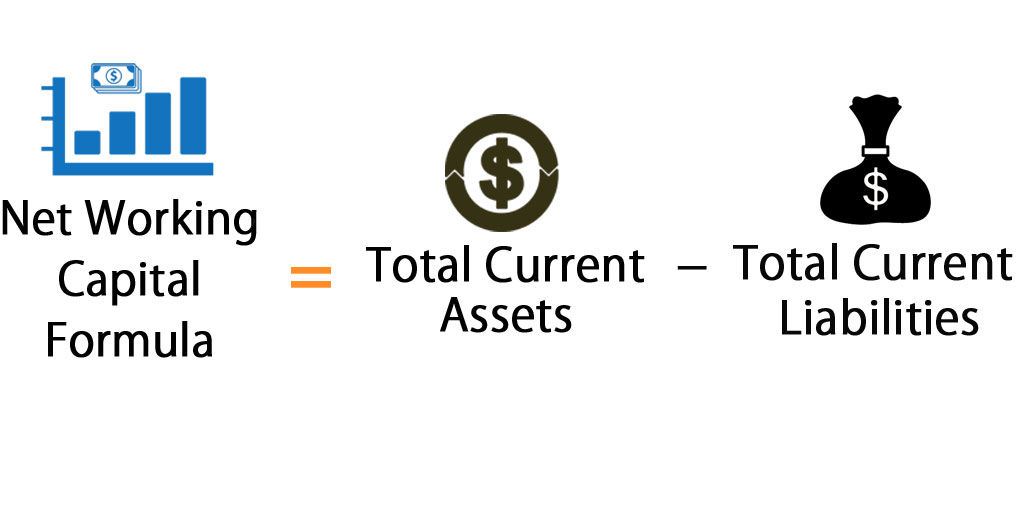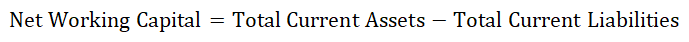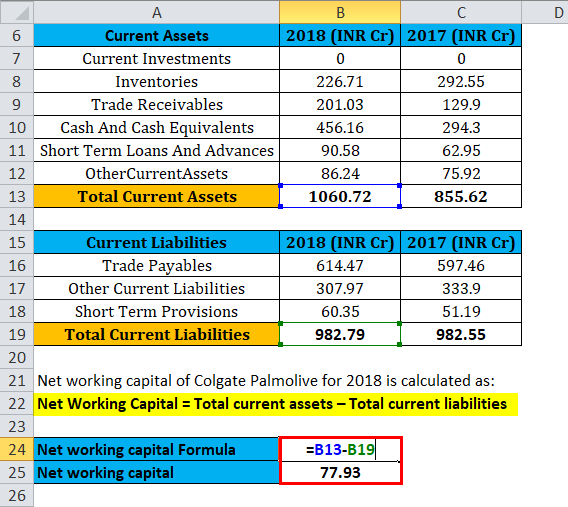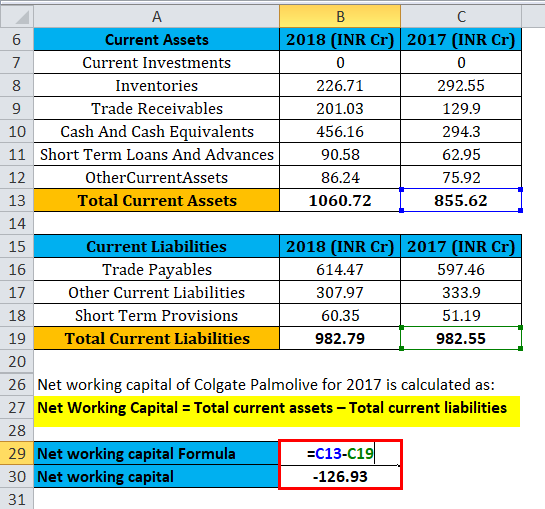# Net Working Capital FormulaNet Working Capital Formula (Table of Contents)

• Net Working Capital Formula
• Net Working Capital Calculator
• Net Working Capital Formula in Excel (With Excel Template)

## Net Working Capital Formula

In simple words, the Net working Capital formula helps in evaluating the short-term liquidity position of the company. The formula for Net working capital is:### Example of Net Working Capital Formula

Let us understand Net working capital with the help of following practical example:

Net Working Capital Formula – Example #1

Consider a company called XYZ ltd that operates in a Retail segment has the following current assets and current liabilities:

Cash: 10000

Accounts receivable: 6000

Inventory: 20000

Accounts payable: 3000

Outstanding salaries: 5000

Following steps need to be followed for calculating Net working capital:

Step 1: Identify the Current Assets and Current Liabilities.

Current assets are any assets that can be converted into cash, typically in a year; whereas Current liabilities are any liabilities that are required to pay in a year.

Current assets in the above example is calculated as:

• Current assets = Cash + Accounts receivable + Inventory
• Current assets = 10000 +6000 + 20000
• Current assets = 36000

Current liabilities in the above example is calculated as:

• Current Liabilities = Accounts payable + Outstanding salaries
• Current Liabilities = 3000 + 5000
• Current liabilities = 8000

Step 2: Net working capital calculation:

Net Working Capital is Calculated using Formula

• Net Working Capital = Total current assets – Total current liabilities
• Net Working Capital = 36000 – 8000
• Net Working Capital = 28000

Since XYZ ltd current assets exceeded the current liabilities, the working capital of XYZ Ltd is positive. This indicates that XYZ Ltd can pay all their current liabilities using only current assets. In other words, the company is highly liquid and financially sound in the short-term. They can use the extra liquidity to grow their business and expand further.

If XYZ Limited’s liabilities would have exceeded the assets, the working capital would be negative indicating that the short-term liquidity is not good, and the current liabilities cannot be paid off with the current assets.

Net Working Capital Formula – Example #2

Now that we got the flair of NWC, let us now go ahead and do a practical Net working capital calculation of Colgate Palmolive – India.

The following figures have been extracted from the financials of Colgate Palmolive – India

 Current Assets 2018 (INR Cr) 2017 (INR Cr) Current Investments 0 0 Inventories 226.71 292.55 Trade Receivables 201.03 129.9 Cash And Cash Equivalents 456.16 294.3 Short Term Loans And Advances 90.58 62.95 Other Current Assets 86.24 75.92 Total Current Assets 1060.72 855.62
 Current Liabilities 2018 (INR Cr) 2017 (INR Cr) Trade Payables 614.47 597.46 Other Current Liabilities 307.97 333.9 Short Term Provisions 60.35 51.19 Total Current Liabilities 982.79 982.55

Let us now calculate Net working capital of Colgate Palmolive – India, based on the above figures.

• Net Working Capital = Total current assets – Total current liabilities
• Net working capital = 1060.72– 982.79
• Net working capital = 77.93 Cr

Based on the above calculation, the Net working capital of Colgate Palmolive – India is positive, which indicates that the short-term liquidity position of the company is positive.

If you compare the liquidity position of Colgate from a previous year, it has improved significantly.

• Net Working Capital 2017 = Total current assets – Total current liabilities
• Net Working Capital 2017= 855.62 – 982.55
• Net Working Capital 2017 = -126.93 Cr

The Net working capital of Colgate Palmolive – India has increased from -126.93 Cr in 2017 to 77.93 Cr in 2018. This means that the liquidity position of the company is better off in 2018 than in 2017.

### Explanation of Net Working Capital Formula

Net working capital is an important measure, that helps the investors in determining the liquidity position of the company. It basically indicates if the company has enough short-term convertible assets to meet its short-term debt obligations.

The Net Working Capital formula can be broken down into two components:

1. The first component of the Net Working Capital formula is Current assets. Current assets are referred to the assets that can be converted into cash, within a year’s time period. Current assets can be Cash, Sundry debtors, Inventory, Accounts receivables etc.
2. The second component of the Net Working Capital formula is Current liabilities. Current liabilities are the debt obligations that need to be paid in a year’s time. Current liabilities consist of Accounts payable, Sundry creditors, Outstanding salaries etc.

It also makes sense to compare the NWC with the previous year’s figures, in order to determine the trend. It indicates whether the company is maintaining its positive/negative liquidity position in a consistent manner. The more positive the trend is, the more liquidated the company is.

While a negative working capital is a bad sign, however, it needs to be noted that a negative working capital does not mean that the company is going to die. It just indicates that the short-term liquidity position of the company is bad. A company can still meet its short-term debt obligations, regardless of the number of current assets it has if the company has easy access to short-term debt, such as a line of credit. If the company enjoys a considerable reputation in the market, it can get easy access to the credit to meet its short-term debt obligations.

### Significance and Use of Net Working Capital Formula

• The Net working capital calculation is mostly done in the investment analysis. An investor before investing in a company, or a lender before lending to the company, usually try to determine the liquidity position of the company by calculating the Net working capital.
• If the Net working capital is positive, the company has enough current assets to pay off its debt. It gives the investor assurance that the company is stable.
• If the Net working capital is negative, the company does not have enough current assets to pay off its short-term debt. Although it’s a poor sign, the investor need not base his decision on the single metric. The investor will further dig in by using other metrics like Profit margins, Asset turnovers to determine the financial health of the company.

To sum it up, Net working capital is a very useful metric that gives out the financial health of the company with a single calculation. However, it is also recommended to consider the other metrics as well and not base the decision on the single metric. Now that you have understood the concept, and gone through the various calculations, it is recommended that you try your hand on Net working capital calculation by doing it practically. A template has been worked out for you, please try to understand and attempt the calculation on your own.

### Net Working Capital Calculator

You can use the following Net Working Capital Calculator

 Total Current Assets Total Current Liabilities Net Working Capital Formula =

 Net Working Capital Formula = Total Current Assets – Total Current Liabilities = 0 – 0 = 0

### Net Working Capital Formula in Excel (With Excel Template)

Here we will do the same example of the Net Working Capital formula in Excel. It is very easy and simple. You need to provide the two inputs i.e Total current assets and total current liabilities

You can easily calculate the Net Working Capital using Formula in the template provided.

First, we calculate the Net Working Capital of Colgate Palmolive for 2018then, First, we calculate the Net Working Capital of Colgate Palmolive for 2017You can download this Net Working Capital Template here – Net Working Capital Formula Excel Template

### Recommended Articles

This has been a guide to a Net Working Capital formula. Here we discuss its uses along with practical examples. We also provide you with Net Working Capital Calculator with downloadable excel template. You may also look at the following articles to learn more –

1. Examples of Capacity Utilization Rate Formula
2. Guide to Operating Profit Margin Formula
3. Calculate Preferred Dividend Using Formula
4. Overhead Ratio Formula

The post Net Working Capital Formula appeared first on EDUCBA.

This post first appeared on Best Online Training & Video Courses | EduCBA, please read the originial post: here

# Subscribe to Best Online Training & Video Courses | Educba

Get updates delivered right to your inbox!

×